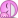# Finding net charge on a sphere's surface

Homework Statement:
We have a thin spherical shell with a spherically symmetric negative charge distribution inside. The radius of the shell is ##R=0.705\,m##, and the electric field strength at its surface is ##E=867\,N/C## everywhere, and is pointing radially toward the center of the sphere.
What is the net charge within the sphere's surface?
Relevant Equations:
.
The electric field caused by the surface distribution on a point ##a## meters far from it is$$E(a)=\frac{kQ}{(R+a)^2}$$from which I get$$Q=\frac{(R+a)^2E(a)}{k}=\frac{(R)^2E(0)}{k}=\frac{(0.705)^2\times867}{8.99\times10^9}\approx4.79\times10^{-8}$$and I take its negative because the direction of the field is inwards.
I'm being told that this is wrong, though. Have I misunderstood the question, or approached it incorrectly?

Steve4Physics
Homework Helper
Gold Member
Hi. Your answer is numerically correct. (I would use a simple '=' sign though.) Your answer is missing the minus sign and unit.

You should use E(0) = -867N/C (radial sign convention), then you get the missing minus sign in the answer. And never forget units!!!

Hi. Your answer is numerically correct. (I would use a simple '=' sign though.) Your answer is missing the minus sign and unit.

You should use E(0) = -867N/C (radial sign convention), then you get the missing minus sign in the answer. And never forget units!!!
Thank you! Yes, I know about the negative sign. ;)
and I take its negative
Are you sure, though? This answer is being rejected in my HW platform.

Steve4Physics
Homework Helper
Gold Member
Hi. Sounds like the HW platform may have a bug or incorrect answer (it happens). Have you tried entering the (incorrect) value without a minus sign? Have you checked any other instructions - e.g. maybe you are meant to enter the answer in nC. Sorry, can't think of anything else.

maybe you are meant to enter the answer in nC
Yes...•Steve4Physics